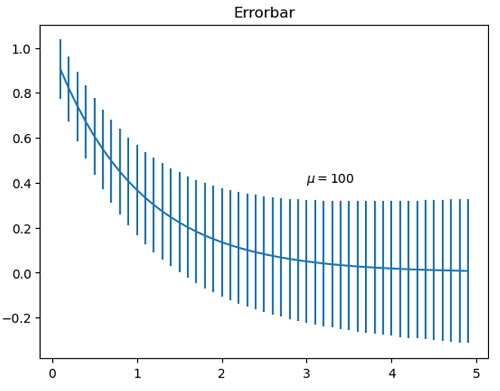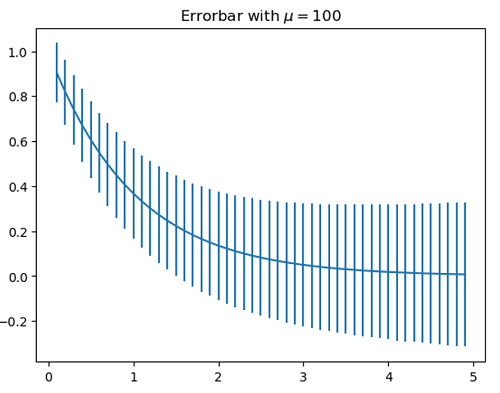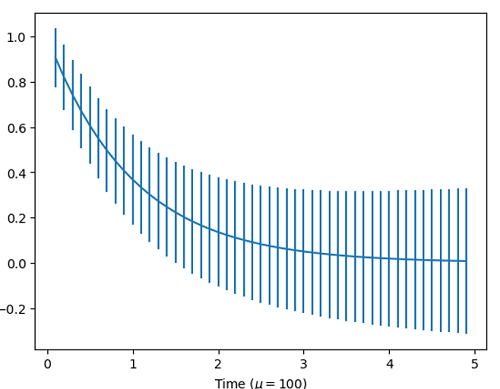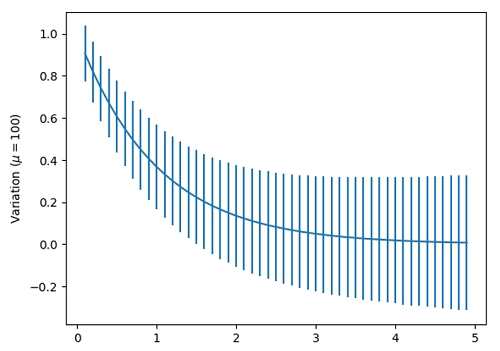# Python | Adding mu in Plot Label

In this article, we are going to learn how to add μ (mu) letter in the plot label in Python?
Submitted by Anuj Singh, on July 14, 2020

μ (Mu) is very often used greek mathematical letters and has a higher repetition in probability. In this article, we are going to add μ using a command in matplotlib.

plt.text(3, 0.4, r'$\mu=100$')plt.title('Errorbar with 'r'$\mu=100$')
#Adding μ in title of the figureplt.xlabel('Time ('r'$\mu=100)$')
#Adding μ in title of the figureplt.ylabel('Variation ('r'$\mu=100)$')
#Adding μ in title of the figure## Python code for adding mu in plot label

import numpy as np
import matplotlib.pyplot as plt

x = np.arange(0.1, 5, 0.1)
y = np.exp(-x)
yerr = 0.1 + 0.1 * np.sqrt(x)

# In text
plt.figure()
plt.errorbar(x, y, yerr=yerr)
plt.title('Errorbar')
plt.text(3, 0.4, r'$\mu=100$')
plt.show()

# In title
plt.figure()
plt.errorbar(x, y, yerr=yerr)
plt.title('Errorbar with 'r'$\mu=100$')
plt.show()

# In x-axis label
plt.figure()
plt.errorbar(x, y, yerr=yerr)
plt.xlabel('Time ('r'$\mu=100)$')
plt.show()

# In y-axis label
plt.figure()
plt.errorbar(x, y, yerr=yerr)
plt.ylabel('Variation ('r'$\mu=100)$')
plt.show()


Output:

Output is as figure


Languages: » C » C++ » C++ STL » Java » Data Structure » C#.Net » Android » Kotlin » SQL
Web Technologies: » PHP » Python » JavaScript » CSS » Ajax » Node.js » Web programming/HTML
Solved programs: » C » C++ » DS » Java » C#
Aptitude que. & ans.: » C » C++ » Java » DBMS
Interview que. & ans.: » C » Embedded C » Java » SEO » HR
CS Subjects: » CS Basics » O.S. » Networks » DBMS » Embedded Systems » Cloud Computing
» Machine learning » CS Organizations » Linux » DOS
More: » Articles » Puzzles » News/Updates